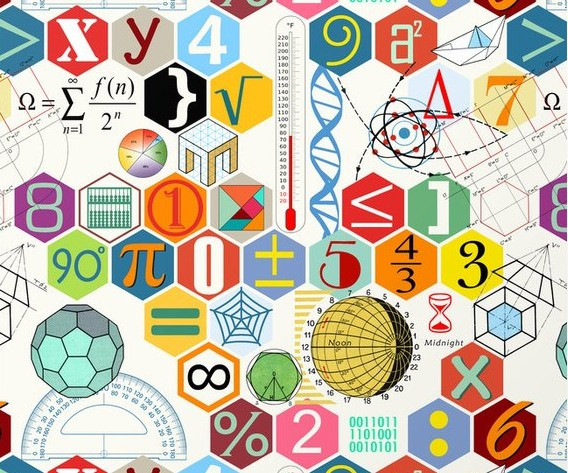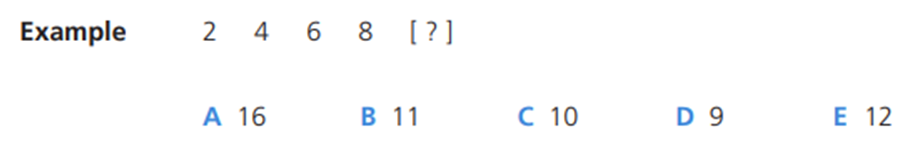Search
•headstart11plus

# How to answer the Verbal Reasoning questions in the 11+ (Blog series 3 – Using Numbers)

Updated: Dec 28, 2021Verbal Reasoning aims to evaluate the ability of a pupil to think constructively rather than just recognise vocabulary. It covers a whole range of question types, however predominantly it is how letters, words or numbers can be used to complete a task. VR is not taught in schools, and puts pupils who do not practice these questions at a disadvantage.

Our blog series on how to answer the Verbal Reasoning (VR) questions in the 11+ continues with the next types of questions pupils may be asked for the VR section of the 11+; Using Numbers. In these questions pupils need to use numbers to complete series, complete equations or find a missing number.

We will be looking at one of the main question types in the using numbers category; number series.

Number Series

In these questions pupils are given a series of numbers. Pupils need to find the number to continue the series in the most sensible way.In this example, you add 2 each time, so the answer will be 10. For other series it may not be as obvious, and you may need to perform different sorts of operations (+, -, x, ÷). There might also be two series involves, where the pattern goes from the 1st number to the 3rd, 5th ,7th, etc. and at the same time there is a pattern going from the 2nd number to the 4th, 6th, 8th, etc.Q1. In this series there is a pattern from the 1st to 3rd, 5th and 7th numbers, as well as for the 2nd, 4th, 6th and 8th. In the pattern of the first, third, fifth numbers they are each increasing one each time. For the pattern for the second, fourth, sixth numbers (the pattern which we need) the numbers are decreasing by one each time. 24 minus 1 will give us 23.

Q2. In this series the numbers are increasing from one number to the next, but in an irregular fashion (4, 3, 4, 3, etc.). The gap from 4 to 8 is four, the gap from 8 to 11 is three, the gap from 11 to 15 is four, the gap from 15 to a8 is three. So if we had a gap of four from 18 we would get 22.

Q3. D – In this series there is a similar jump as there was in Q1, however this time the gap each time is 3 (28 to 25, to 22, etc.). The gap we need is with the 2nd, 4th ,6th numbers and that is 5 (32 to 27, to 22, to 17, and then 17 minus 5 would give us 13.

Q4. This is probably the easiest of all the questions. The gap between the numbers is increasing by one each time. The gap from 20 to 23 is 3. The gap from 23 to 27 is 4. The gap from 27 to 32 is 5. The gap from 32 to 38 is 6. So we can reasonably assume that we will need a gap of 7 from 38 to our number, and that would mean our answer is 45.

Please note that these are really simplified examples of the questions pupils will receive on the 11+. We have a wealth of experience of assisting pupils prepare for all aspects of the secondary transfer test (11+), including Verbal Reasoning.